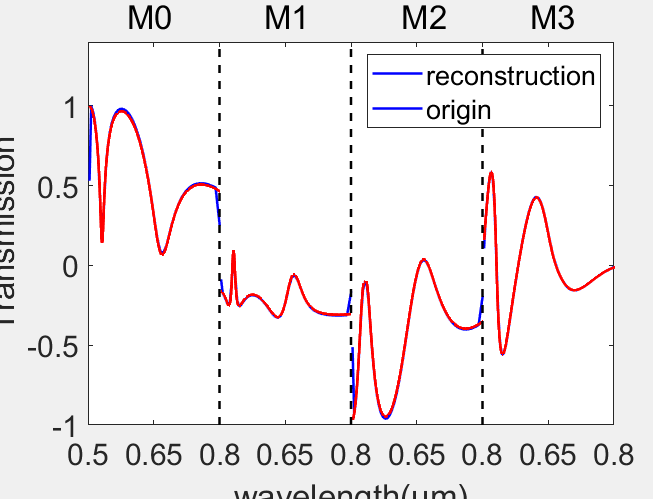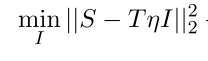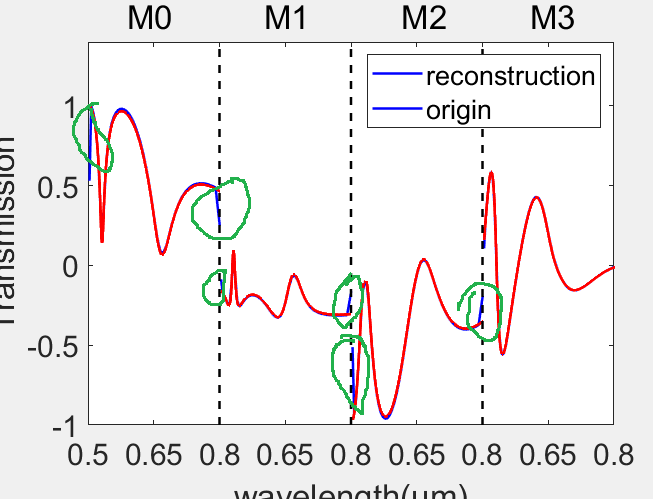# Question: How to use CVX to solve some discontinuous problems, is this a problem CVX can solve or is it just a mathematical problem?

The object to be optimized is a discontinuous function, which can lead to serious drags in the optimization results, this picture shows the function I need to get from CVX.the red line is ORIGIN and the blue one is RECONSTRUCTION , We can see that there is a drag at the break point.

cvx_begin
variable x(n);
minimize(norm(S-T*x))
subject to
cvx_end

and for X is the discontinuous function that I want to restore, S and T is what I know.

I doubt anyone can tell you unless you specify the mathematical optimization problem you want to solve.

Recall the problem should be a (mixed-integer) convex optimization problem.

the problem is very simple there is S=T * I ,We know T and S so want to get the I, always the T is not a n*n matrix so I can’t solve it by T inversion .is that a linear least squares problem? I don’t know, because I don’t understand what all your symbols are. But how would a linear least squares model match the curves you plotted? So you need to clarify what all the symbols are.

Maybe I need to clear my question again, in the beginning with T (m * n) and I (n * 1), we get S = T * I (this is usually a unknown physical process, but we know T matrix, and then we can get S in some ways ). so in fact, we know that S(m * 1) and T(m * n), need to work out the I(n * 1), we use CVX tools, but because of I is a discontinuous vector, The resulting I has some drags at the discontinuity point(The part that comes out of the green coil).It is still not clear to me what you want. Maybe some type of mixed-integer least squares which will result in MIQP or MISOCP. See for instance, https://yalmip.github.io/example/modellingif/ or https://math.stackexchange.com/questions/2400829/mixed-integer-conditional-least-squares-regression . But if you do it in CVX, you will have to “manually” implement the logic, rather than relying on higher level constructs such as YALMIP’s `implies`. You can see some “manual” modeling in “9.2.3 Convex piecewise linear regression” of https://docs.mosek.com/modeling-cookbook/mio.html#mixed-integer-conic-case-studies .